### RESULTS: Angular Kinematics

Segment angle: In the chin-up and the pull-up the angular motions were a little different (as represented by the two graphs below). A major difference was the hand grip position in both movements. The maximum clockwise angle for the trunk in the chin-up was &endash;8 degrees. The minimum angle was &endash;33 degrees for the trunk. The maximum counterclockwise angle for the trunk in the chin-up was &endash;33 degrees. The minimum angle was &endash;8 degrees for the trunk. The range of motion for the trunk segment in the chin-up was 25 degrees. The maximum clockwise angle for the trunk in the pull-up was &endash;11 degrees. The minimum angle was &endash;29 degrees for the trunk. The maximum counterclockwise angle for the trunk in the pull-up was &endash;29 degrees. The minimum angle was &endash;11 degrees. The range of motion for the trunk segment in the pull-up was 18 degrees. The total range of motion of the trunk was greater in the chin-up than in the pull-up.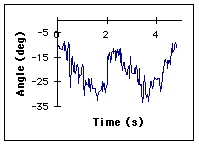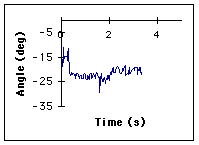Figure 3. Trunk angle during the chin-up (left) and the pull-up (right). The trunk angle is calculated from the vertical axis about the hip joint. Anatomical position corresponds to zero degrees. Increasing values represent clockwise rotation.

Joint angle 1. In the chin-up and the pull-up the angular motions of the elbow joint were slightly dissimilar. During the chin-up the maximum flexion angle was 52 degrees. The maximum extension was 178 degrees. The range of motion was 126 degrees. During the pull-up the maximum flexion angle was 32 degrees. The maximum extension angle was 168 degrees. The range of motion was 136 degrees. The total range of motion of the elbow joint in the pull-up was greater than in the chin-up.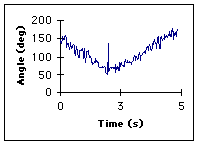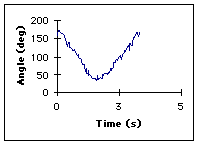Figure 4. Elbow joint angle during the chin-up (left) and the pull-up (right). Elbow joint angle is calculated as the angle between the forearm and the arm. Anatomical position corresponds to 180 degrees. Increasing values represent extension.

Joint angle 2. The angular motion of the shoulder joint angle in both movements was different. For the pull-up, the maximum shoulder flexion angle was 212 degrees, while the maximum extension angle was 394 degrees. The total range of motion for the pull-up was 182 degrees. For the chin-up, the maximum shoulder flexion angle was 199 degrees, while the maximum shoulder extension angle was 362 degrees. The total range of motion for the chin-up was 163 degrees. The total range of motion of the shoulder joint was greater in the pull-up than in the chin-up.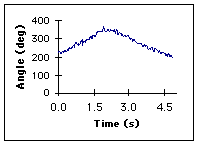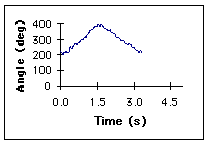Figure 5. Shoulder joint angle during the chin-up (left) and the pull-up (right). Shoulder joint angle is calculated as the angle between the arm and the trunk. Anatomical position corresponds to 360 degrees. Increasing values represent extension.

Joint velocity. When comparing our elbow joint angular velocities, we noticed that the average velocity for the pull up were almost twice as fast as the average velocity for the chin-up. In the chin up the maximum flexion velocity was &endash;36 degrees/sec. The maximum extension velocity was +38 degrees/sec. In the pull up the maximum flexion velocity was &endash;87 degrees/sec, while the maximum extension velocity was +72 degrees/sec.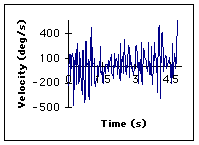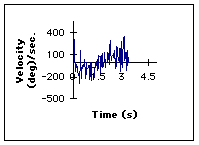Figure 6. Elbow joint angular velocity in the chin-up (left) and the pull-up (right). Positive values represent extensor velocity.

Angle-Angle Plot. Looking at our two angle-angle graphs, we see that our two movements were very coordinated. Each graph consists of a relatively straight diagonal line that represents the coordinated movements. In the plot for the chin up, since it is diagonal this tells us that shoulder extension/flexion and elbow extension/flexion were coordinated. As the shoulder flexes, the elbow extends. As the shoulder extends, the elbow flexes. However, a small loop in the graph could be due to a slight pause in one of the motions of the elbow or shoulder. It could also be due to any rigid or jerky movements. In the pull up plot, there are no loops observed and we see a very straight, diagonal line. This means that as the shoulder flexes, the elbow extends. As the shoulder extends, the elbow flexes. This movement was more coordinated than the chin up.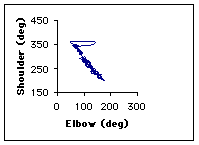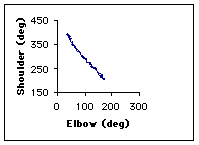Figure 7. Coordination of elbow angle and shoulder angle in the chin-up (left) and pull-up (right). Arrow tails represent the start of the movement and point in the direction that the motion proceeds.